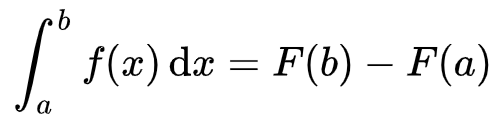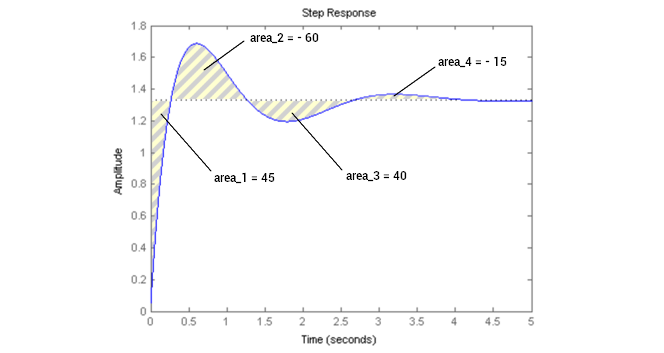# PID: The I, as in integral (How a PID works, part 3)

We go on with the PID letters. This post deals with the I. You can visit the first posts of this serie, the introduction to PID and the proportional post.

Inthe previous post, I introduced to you what was a PID control, and how the P — for proportional — was working in it. This post is about the I of PID.

Welcome to the I-post.

As you can remember from my previous posts, a PID is most of the time a coded feedback loop control working into a system which includes at least:

• a powered actuator (eg. a DC motor),
• a sensor (eg. a speed sensor), and,
• the associated command (most of the time an electronic board with a microprocessor).

Basically, a PID is a tool used for politely but firmly asking a machine to move or react exactly the way you want, while adapting to its changing environment.

The changing environment can be, for example, a dynamic charge, or something slowing the rotation.

I remind you the must-known vocabulary you will need in order to go on reading:

• Set-point: the user-defined value to be reached by the actuator (a speed, a torque, etc.).
• Process variable: the real actuator’s value, retrieved by the sensor in real time.
• Error: the difference between the set-point and the process variable.
• Steady state: when the system stops oscillating and finds its full balance.

Unlike the proportional part of a PID, the integral part can’t be used alone, as it wouldn’t have any effect. Instead of that, integral is either used along with proportional (PI), or with proportional and derivative (PID). The proportional part P is always used in a PID control.

Note: P, PI, PD and PID are the 4 possible combinations of a loop control.

Now, to the point:

The I stands for integral, because it integrates the computed error into the PID command.

What does that even mean?

In the last post, the P-post, you thought you casually took the computed error and that you multiplied it by a chosen constant, P. But what you really did is that you actually multiplied P by the last calculated error. At each loop, its value actually changes:

• The system is running, the process variable evolves at each loop.
• The set-point stays the same, however, unless you want to change it.
• The error, which is equal to `ProcessVariable - setpoint`, evolves as well at each loop.

Then the error must not be seen as a variable anymore, but as a function of time. Let’s call it `ε(t)`.

Let’s get back to the integral thing. Integration is a mathematical operation applied to a function continuous into a given interval. Let me write the method of calculation down here, just for fun:Some people love these strange writings

`f(x)` is the function, and it’s integrated from `x = a` to `x = b`. You can express it like this: “The integral of `f(x)` from a to b is equal to the primitive `F` of `f` at b minus the primitive `F` of `f ` at a. The primitive of a function is the inverse of the derivative, but at this point you don’t really care, we don’t want to go too deep into mathematics. Let’s just say that integrating a function is like calculating the area between its representative curve and the x-axis (abscissa), and into a wanted interval where the function is defined:

Fig: Thank you Wikipedia.org. In this example the interval is [0;a].

Thinking of area, integrating a function — although it’s not too difficult if you know how to use the method — may seem like a pain: it’s like dividing the area into a bunch of really thin rectangles (the thinner the better), calculating their area, and adding them together. It results only in an approximation, because the upper side of a rectangle doesn’t quite follow a curve:

Fig: Each red rectangle has a smaller side which length is dx. Aaand… you can forget it.

There is virtually an infinite number of values stuck between the two numbers 0 and `a`, for example, whatever is `a`. So no one reasonable will accept to do the maths with an almost infinite numbers of thin rectangles.

Let’s breath a moment. There is not only math in life. There is nature too.

There, there.

And today we are lucky: we are writing a PID in programming code, meaning that all the values of the error function `ε(t)` during a set of loops are known and can be written down in a finite number of them, as many as the times the loop iterates. That means the error function `ε(t)` is discretized.
No. Infinite. Number. No. Complicated. Math.

Still the question remains: How to integrate `ε(t)`?
It’s easy: You add together all the previous values of the error function. It’s like adding all the thin rectangles under the error curve.
Then you multiply the total by a constant called `KI`, that will allow you to have a control over the power of the integral part. For example, if `KI` is very small, like `KI = 0.005`, the integral part will have very few effects on the command. `KI`, like `KP`, is a gain you will want to set in order to tune your final PID.

Here is how you want to express it: you declare a variable, let’s call it `sum_e`, that will iterate with each new value of the error `ε(t)` at each loop of the system, something like `sum_e += e` (`e` being the error at each loop), and you multiply this variable by `KI` in the command function. We now have this:

Fig: First, the original PID command.

Fig: Then, the actual integral function of the error.

Fig: Last, the PID command with Proportional and Integral parts. Beautiful.

Visually, after many loops, the operations made by the integral command look like this on the system:Unit of the areas are in cherry-pie

In this example, the expression of `fI(e)` at `t = 4.5s` is `fI(e) = KI*(45 -60 + 40 -15) = 10*KI`

It’s almost time for you to implement the integral part into your code. But before you do that, let’s figure out what is the effect of the integral part on a feedback loop control.

## The effects of I

With the integral part, it’s as if the command had a memory: it knows the previous errors that were made. And the bigger the sum of the previous errors (`sum_e` ), the bigger `fi(e)`. The bigger `fi(e)`, the stronger the command. So if the past errors were too important before, it’s time to correct with a bigger command.

• `ε(t)` being a function of time, you can see that every single value it took in the past loops has an effect on the integral part of the PID:

The integral looks into the past of the system.

• Adding an integral command will correct the static error induced by a proportional command set alone. Indeed, as long as there is an error, i.e. `ε(t) ≠ 0`, even if `ε(t)` is small enough to have no effect to the proportional part, it will still impact the system with its residual value.

You now have two parameters to tune, `KP` and `KI`. You can program a proportional control for your system, and refine it with an integral control, which makes it a PI.
In the next post, we will see the last letter of PID, the D.

To summarize:

• The integral command adds together all the previous errors of the system and multiplies it with a factor `KI`.
• An integral command can’t be used alone; it must be used at least with a proportional command (PI), or with a full PID command.
• It corrects the static error, or steady-state error, that appears with a proportional command alone, and fine-tunes the whole system through time.
• In general, the integral part is making the system more precise.